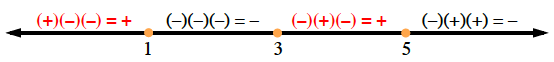### Home > PC3 > Chapter 4 > Lesson 4.3.2 > Problem4-119

4-119.

Solve the inequalities below and express the solutions in both interval and inequality notation.

1. $(1-x)(x-3)(x-5)>0$

The $>$ sign means that the three factors, when multiplied, are positive.
Check a value in each interval to determine the positive intervals.1. $x^2-2x-15<0$

1. Factor and determine the boundary points.
2. Sketch a number line and test your intervals.